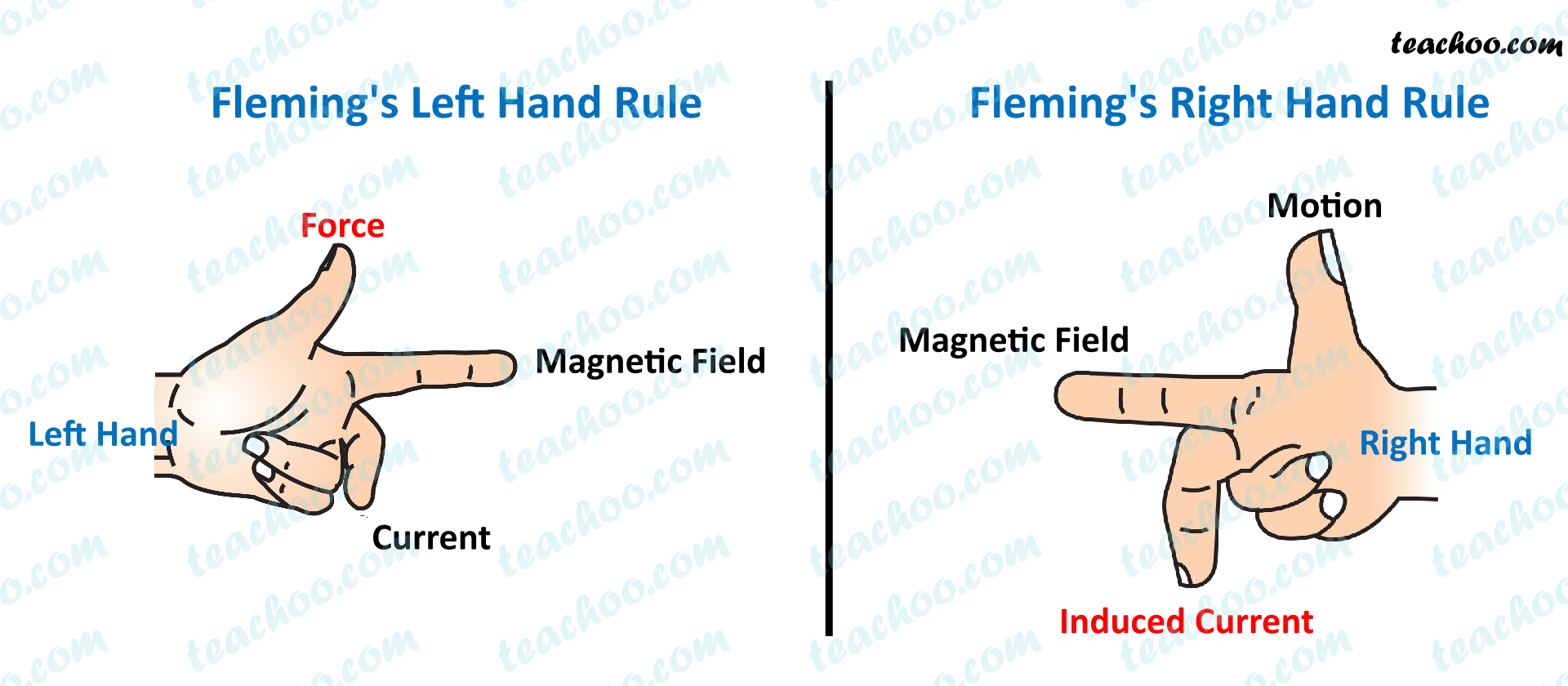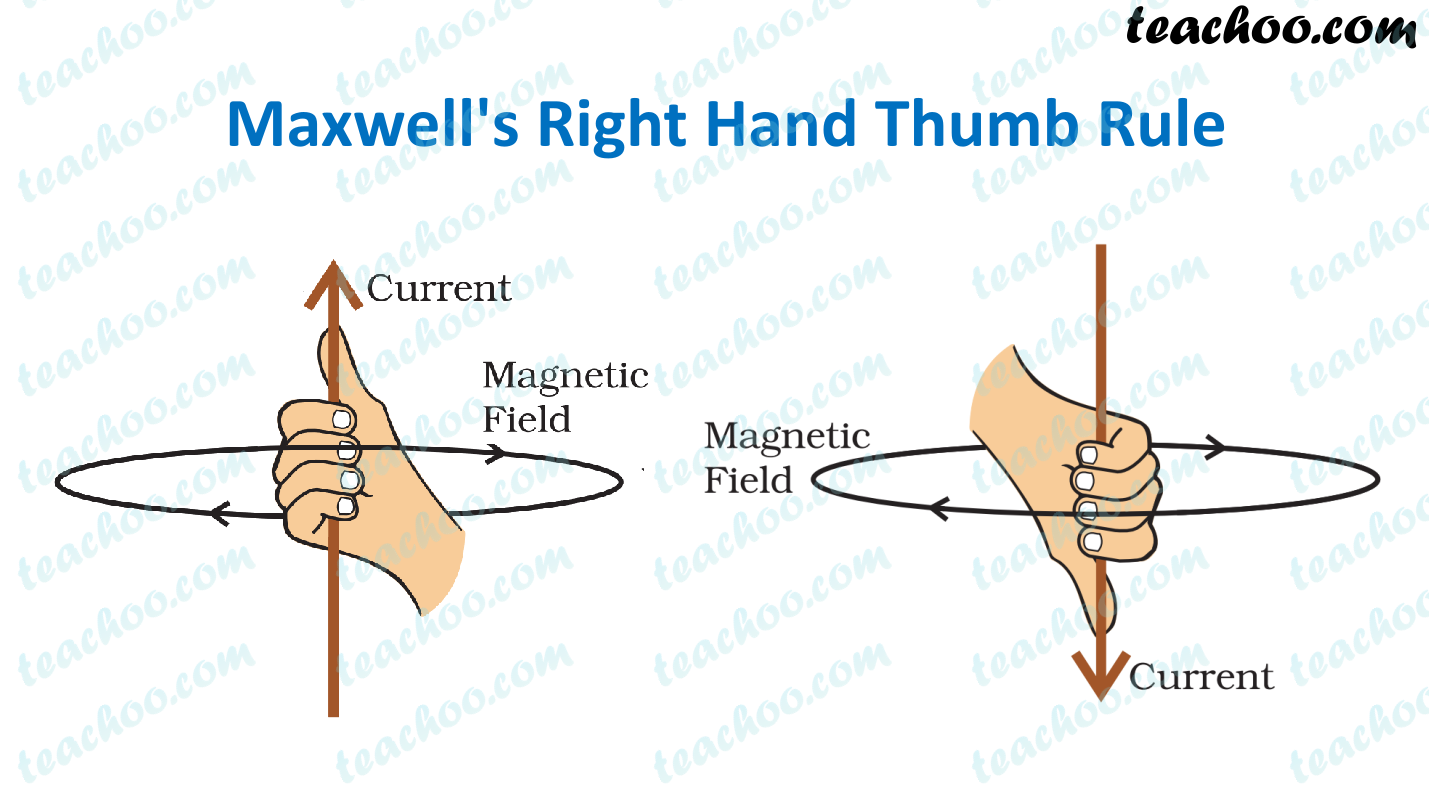Concepts

Class 10
Chapter 13 Class 10 - Magnetic Effects of Electric CurrentRight hand thumb rule Fleming's Left hand rule Fleming's Right hand rule Used to find direction of magnetic field Used to find the direction of force/motion of conductor Used to find the direction of the induced current Curl of our fingers determines the direction of magnetic field Thumb determines the direction of motion (Force) Middle finger determines the direction of induced current If we point the thumb of our right hand in the direction of the current, Then the direction which our fingers curl gives the direction of the current. Fleming’s left-hand rule states that if we hold the forefinger, the middle finger and the thumb of our left hand at right angles to one another such that Forefinger points in the direction of magnetic field Middle finger in the direction of the current Then the direction which the thumb points gives the direction of the force/motion of the conductor. Fleming’s right-hand rule states that if we hold the forefinger, the middle finger and the thumb of our right hand at right angles to one another such that Forefinger points in the direction of magnetic field Thumb in the direction of the motion of conductor Then the direction which the middle finger points gives the direction of the induced current. - Current is present in the circuit There is no power source. Current is induced in the circuit - Force is produced Force is applied It is used to find Direction of Motion in Electric Motors It is used to find direction of Induced Current in Electric Generators

Maxwell's Thumb Rule looks like the below figureLearn in your speed, with individual attention - Teachoo Maths 1-on-1 Class